# 安卓自定义View——Matrix

### 1、Matrix简介

1. Matrix是一个矩阵，主要功能映射视图中的坐标，这里的映射有两重意思，一是将坐标以矩阵的形式表示，类似于数学中的向量，二是映射手机屏幕坐标和安卓坐标的对应关系，在物理屏幕中坐标原点为左上角（0，0）处，而在Android了开发中使用的坐标是去除状态栏和导航栏的高度，即（0，0）位置在状态栏之下，假设状态栏30px，导航栏60px，则Android中的（0，0）对应物理坐标（0，90）
2. 矩阵除了映射坐标外还存在另一个作用，数学中都学过矩阵的变换，通过矩阵变换实现向量的修改，同样思想如果把整个变换作用于某个图形的所有像素，则可以实现图形的变换，这就是Matrix的另一个功能，实际他一直在默默的做事情

### 2、Matrix的矩阵形式

• 矩阵公式：View的属性及其改变都可以映射其中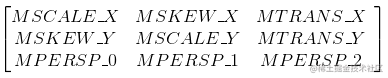1. MSCALE_X、MSACALE_Y：代表View的X、Y坐标的缩放系数；
2. MTRANS_X、MTRANS_Y：代表View的X、Y的方向的位移量；
3. MSKEW_X、MSKEW_Y：代表View的X、Y的方向的位侧错切；
4. MSCALE_X、MSACALE_Y、MSKEW_X、MSKEW_Y：共同决定View的旋转；
5. MPERSP用于控制透视，通常为（0，0，1）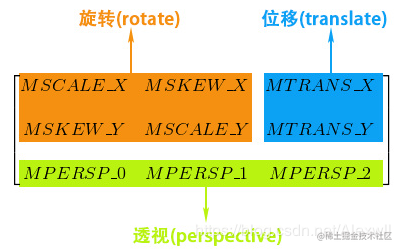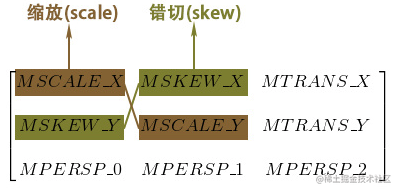View的改变和动画执行的背后都是在做Matrix的计算，通过一定规则改变Matrix矩阵的值，将改变后的数据反应在视图上就行成了View的展示，所以在自定义View的过程中可以将很多关于坐标和动画的过程用Matrix实现，不过安卓提供了很多方法简化了功能，将Matrix推至幕后工作。

• 矩阵计算规则
1. 矩阵乘法不满足交换律，即 A*B ≠ B*A
2. 矩阵乘法满足结合律，即 (A*B)*C = A*(B*C)
3. 矩阵与单位矩阵相乘结果不变，即 A * I = A

3、使用Matrix实现View动画

• 缩放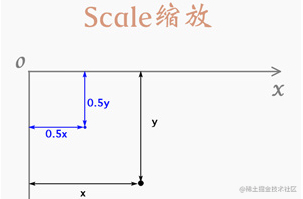``````x = k1 * x0
Y = k2 * y0 复制代码``````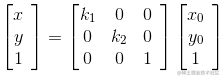• 位移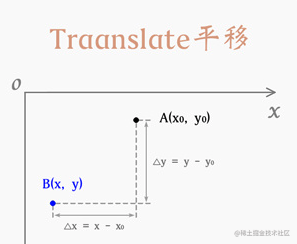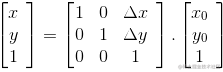• 旋转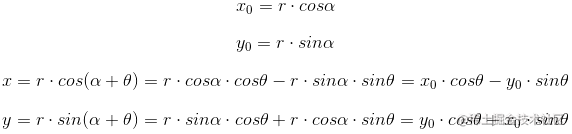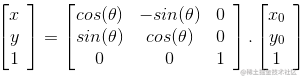• 错切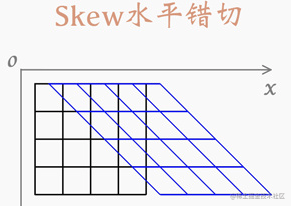``````x = x0 + k * y0
Y = y0复制代码``````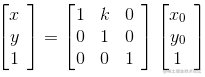### 3、Matrix的方法和使用

• preXxx（）：矩阵前乘 M * S （ 相当于矩阵右乘），通过乘积得到改变结果矩阵，系统中提供了集中封装好的处理方法
1. preTranslate(float dx, float dy)：矩阵位移，修改单元矩阵中MTRANS_X、MTRANS_Y后相乘
2. preScale(float sx, float sy):缩放功能；右乘缩放矩阵
3. preRotate(float degrees, float px, float py)：旋转功能；乘旋转矩阵
4. preSkew(float kx, float ky)：错切；乘错切矩阵
``````val bitmap = BitmapFactory.decodeResource(context.resources, R.mipmap.ic_launcher)
canvas?.drawBitmap(bitmap, matrix, paint) //绘制基本的Bitmap

canvas?.translate(0f, 200f)
val matrix = Matrix()
matrix.preScale(2f,2f) //缩放2倍
canvas?.drawBitmap(bitmap, matrix, paint)

canvas?.translate(0f, 300f)
val matrixT = Matrix()
matrixT.preRotate(90f) //旋转90度
canvas?.drawBitmap(bitmap, matrixT, paint)

canvas?.translate(0f, 300f)
val matrixR = Matrix()
matrixR.preTranslate(100f,0f) //位移
canvas?.drawBitmap(bitmap, matrixR, paint)

canvas?.translate(0f, 300f)
val matrixS = Matrix()
matrixS.preSkew(0.5f,0f) //X轴方向错切
canvas?.drawBitmap(bitmap, matrixS, paint)复制代码``````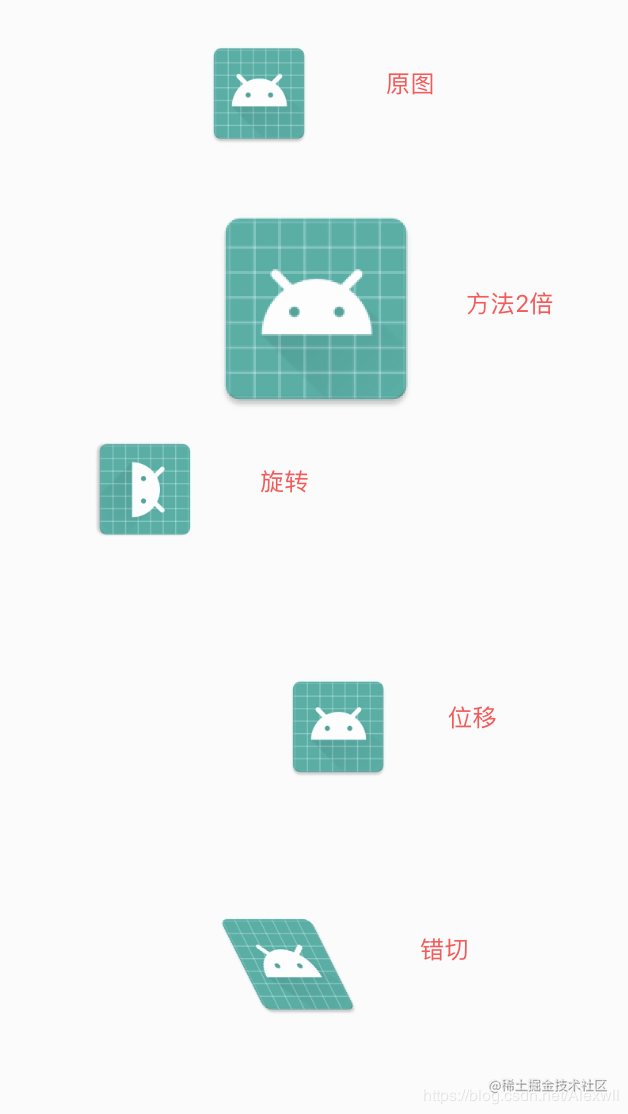• postXxx（）：矩阵后乘 S * M（相当于左乘）
1. postTranslate(float dx, float dy)：位移
2. postScale(float sx, float sy):缩放
3. postRotate(float degrees, float px, float py)：旋转
4. postSkew(float kx, float ky)：错切
5. 使用方法和上面Pre一致
• 组合使用
1. 使用post和pre可以实现同样的效果，具体效果取决最终的表达式；
``````val matrix = Matrix()
matrix.postScale(2f, 2f) // S * M
matrix.postRotate(100f)  // R * ( S * M ) = R * S * M = R * S

val matrix = Matrix()
matrix.preRotate(100f) //  M * R
matrix.preScale(2f,2f) // M * R * S = R * S (与上面一致)复制代码``````1. 所有的操作(旋转、平移、缩放、错切)默认都是以坐标原点为基准点的，如果想指定原点需要借助平移实现
2. Matrix的操作是改变坐标系的，每次操作的坐标系状态会保留，并且影响到后续状态，如果不需要对后需的操作影响，需要重置Matrix
• 实现基于设置的某一点旋转
1. 旋转默认中心为坐标起点，现在我们想设定可以围绕制定点（x,y）旋转，分析一下实现的过程，首先将坐标以（x,y）为原点这样默认的中心就是（x,y）即需要将坐标系位移（x,y），然后进行旋转，此时的View是以（x,y）中心旋转，旋转后此时的坐标系与开始相比位移了（x,y），此时只需平移回去即可，按照此流程程序的伪代码应该为： M * T * R * （-T） = T * R * -T
``````Matrix matrix = new Matrix();
matrix.preTranslate(pivotX,pivotY);
matrix.preRotate(angle);
......
matrix.preTranslate(-pivotX, -pivotY);复制代码``````1. 上面的写法虽然实现克功能，但将对坐标系平移的操作放在了两端，中间夹杂着转换操作，使用post变换写法使转换和坐标系的平移分离，代码如下，效果一样：T * R * -T
``````Matrix matrix = new Matrix();

// 各种操作，旋转，缩放，错切等，可以执行多次。

matrix.postTranslate(pivotX,pivotY);
matrix.preTranslate(-pivotX, -pivotY);复制代码``````• pre和post使用注意点：
1. pre和post都可以实现同样效果，矩阵最终的效果取决于最后的乘积顺序，但使用时要注意尽量先选择一种设置好效果后，在根据场景优化即可；
2. 上面的各种pre和post时针对单元矩阵操作的，所以可以有很多同样效果的变换，但当操纵不是单元矩阵时要小心对待，矩阵不满足交换律
• equals()：比较两个Matrix的数值是否相同
• toString（）：将Matrix转换为字符串（对象集合的形式输出）
• toShortString（）：将Matrix转换为字符串
``````Log.e("=======",matrix.toString())
Log.e("=======",matrix.toShortString())

2019-04-09 13:11:05.292 12097-12097/? E/=======: Matrix{[2.0, 0.0, 0.0][0.0, 2.0, 0.0][0.0, 0.0, 1.0]}
2019-04-09 13:11:05.292 12097-12097/? E/=======: [2.0, 0.0, 0.0][0.0, 2.0, 0.0][0.0, 0.0, 1.0]复制代码``````• set(Matrix src)：设置新的Matrix
• reset（）：重置Matrix
• setValues(float[] values)：相当于赋值Matrix，根据提供的集合提取数据到Matrix中；
1. values数据长度必须大于等于9，截取前面9个数据为Matrix
``````val values = floatArrayOf(2.0f, 0.0f, 0.0f,0.0f, 2.0f, 0.0f,0.0f, 0.0f, 1.0f)
matrix.setValues(values)

//效果相当于：matrix.preScale(2f,2f)复制代码``````• getValues（float[] values）：获取Matrix中的数值保存在values中，与setValues（）相对应
• mapPoints：数值计算
1. void mapPoints (float[] pts)：将传入的参数pts经Matrix变换后保存在pts中
2. void mapPoints (float[] dst, float[] src)：将传入的参数src经Matrix变换后保存在dst中
3. void mapPoints (float[] dst, int dstIndex,float[] src, int srcIndex, int pointCount)：可指定一步分数据进行运算
``````float[] pts = new float[]{0, 0, 80, 100, 400, 300};
Matrix matrix = new Matrix();
matrix.setScale(0.5f, 1f);  //将X轴坐标缩小一倍
matrix.mapPoints(pts); //输出pts中结果：[0.0, 0.0, 40.0, 100.0, 200.0, 300.0]复制代码``````• mapRect（）：将rectF中数据计算并将结果保存在Matrix中，并判定是否为矩形，矩形返回true，否则返回false
``````RectF rect = new RectF(400, 400, 1000, 800);
boolean result = matrix.mapRect(rect);• mapVectors：测量向量
1. 使用方式与mapPoints（）一致，只是mapVectors不受位移影响（位移时向量的矢量不变）
``````float[] src = new float[]{1000, 800};
float[] dst = new float;

Matrix matrix = new Matrix();
matrix.setScale(0.5f, 1f);
matrix.postTranslate(100,100);

matrix.mapVectors(dst, src); //输出结果：[500.0, 800.0] ，平移无效
Log.i(TAG, "mapVectors: "+Arrays.toString(dst));

matrix.mapPoints(dst, src); //输出结构：[600.0, 900.0]
Log.i(TAG, "mapPoints: "+Arrays.toString(dst));复制代码``````• setPolyToPoly（）：设置多边形变换，方法不太好理解，简单的说就是通过setPolyToPoly（）将试图的几个点订（图钉）在某个位置，其余未操作的点是可以自由随之变动的
1. boolean setPolyToPoly(float[] src, int srcIndex, float[] dst, int dstIndex, int pointCount)：参数

（1）src：原始数组 src [x,y]，存储内容为一组点

（2）srcIndex：原始数组开始位置

（3）dst：目标数组 dst [x,y]，存储内容为一组点

（4）dstIndex：目标数组开始位置

（5）pointCount：控制点数量：0～4；设置控制点越多，则可操作性越大

• pointCount最多控制4个：

（1）控制一个点：一张纸一个图钉智能实现评移效果，订在哪是哪

（2）控制两个点：实现旋转功能；一张纸两个钉可以将纸斜着钉

（3）控制三个点：实现错切

（4）控制四个点：千奇百怪的图形

• 使用实例（控制四个点，将三个点保持不变，然后修改第四个点位置，图形随之改变）
``````bitmap = BitmapFactory.decodeResource(context?.resources, R.drawable.image)

val src = floatArrayOf(0f,0f,  //原始定点位置数组
bitmap!!.width.toFloat(),0f,
bitmap!!.width.toFloat(),bitmap!!.height.toFloat(),
0f,bitmap!!.height.toFloat())

val dst = floatArrayOf(0f,0f,
bitmap!!.width.toFloat(),0f,
bitmap!!.width.toFloat() - 1500 ,bitmap!!.height.toFloat() - 800,  //修改目标集合的坐标值
0f,bitmap!!.height.toFloat())

matrixPaint.setPolyToPoly(src,0,dst,0,4)   //设置源数据集合、目标集合、控制点个数
matrixPaint.postScale(0.3f,0.3f)   // 设置缩放系数复制代码``````1. 使用效果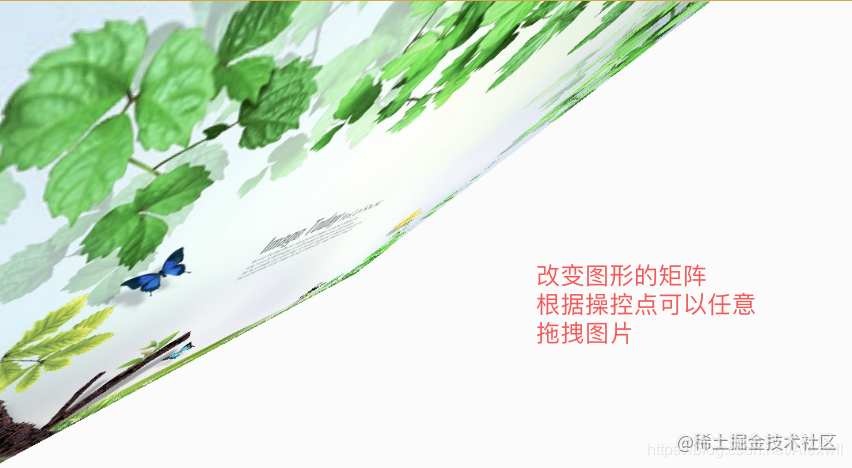• setRectToRect( RectF src, RectF dst, ScaleToFit stf )
1. 将内容从原数据区域填充到目标区域并设置填充模式
2. 提供四种填充模式：CENTER、START、END、FILL
• 矩阵方法
1. invert ：求逆矩阵（乘积未单元矩阵）
2. isIdentity：判断矩阵是否为单位矩阵

### 4、Matrix Camera

• 作用：使用Camera和3D坐标系构建立体效果
• 3D坐标系：坐标系使用中需要注意一下两点
1. Camera坐标系采用左手坐标系，X轴正向向右，Y轴正向向上，Z轴垂直屏幕向里；
2. Camera的坐标系和Matrix及Canvas坐标系Y轴方向相反，Y轴向上为正向
• 三维投影
1. 正交投影：正视图、侧视图、俯视图
2. 透视投影：当View和摄像机在同一直线上时，沿Z轴位移的效果：近大远小、当View和摄像机不在同一直线上时，沿Z轴位移的效果：在缩小的同时靠近摄像机的投影位置（视线相交）
• 常用方法
1. camera.save（）：保存Camera状态，使用方法和Canvas.save()一样，在执行操作前保存Camera
2. camera.retore（）：回滚Camera状态
3. getMatrix（）：计算Camera当前状态下的矩阵状态，并将计算结果赋值到Matrix中
4. applyToCanvas（）：计算Camera当前状态下的矩阵状态，并将计算结果赋值到Canvas中
• translate (float x, float y, float z)：平移
1. X轴方向平移，同向效果一致
``````camera.translate(x, 0, 0);
matrix.postTranslate(x, 0);复制代码``````1. X轴方向平移，反向效果相反
``````Camera camera = new Camera();
camera.translate(0, 100, 0);   //Camera沿Y轴正向位移100，向上

Matrix matrix = new Matrix();
camera.getMatrix(matrix);  //获取当前位置的矩阵信息（移动后的信息）
matrix.postTranslate(0,100); //Matrix沿Y轴正向位移100 （互相抵消，回到原点）复制代码``````1. Z轴平移

（1）、当View和摄像机在同一直线上时，沿Z轴位移的效果：近大远小

（2）、当View和摄像机不在同一直线上时，沿Z轴位移的效果：在缩小的同时靠近摄像机的投影位置（视线相交）

• void rotate (float x, float y, float z)：旋转
1. 旋转中心默认为坐标原点（图片左上角），可使用Matrix改变旋转中心
2. 提供3种直接旋转方法
``````void rotateX (float deg);
void rotateY (float deg);
void rotateZ (float deg);

matrix?.postTranslate(centerX.toFloat(), centerY.toFloat()) //利用Matrix设置旋转的中心
matrix?.preTranslate(-centerX.toFloat(), -centerY.toFloat())复制代码``````• setLocation (float x, float y, float z)：设置相机位置（默认 0，0，-8）
1. 相机和View的z轴距离不能为0，否则无法体现出Z轴效果，正如一叶障目不见泰山一样
2. 摄像机平移一个单位等于View平移72个像素
3. 摄像机右移等于View左移
``````camera.setLocation(1,0,-8);
camera2.translate(-72,0,0);  //二者相等复制代码``````• 实现三维旋转
1. 分析实现思路：继承Animation，在处理动画中根据差值器的执行计算当前的旋转角度，使用camera执行旋转动画和位移动画，最后设置坐标系的平移和恢复
``````class RotateAnimation : Animation {

var centerX = 0 //设置旋转的中心坐标
var centerY = 0
var fromDegree = 0f //设置开始和结束的角度

var toDegree = 0f //设置旋转的角度
var translateZ = 0f //Z轴旋转
var currentDegree = 0f //当前角度

val camera = Camera()
var matrix: Matrix? = null

constructor(centerX: Int, centerY: Int, fromDegree: Float, toDegree: Float, translateZ: Float) {
this.centerX = centerX
this.centerY = centerY
this.fromDegree = fromDegree
this.toDegree = toDegree
this.translateZ = translateZ
}

override fun applyTransformation(interpolatedTime: Float, t: Transformation?) {
super.applyTransformation(interpolatedTime, t)
//根据差值器执行进度计算当前角度
currentDegree = (toDegree - fromDegree) * interpolatedTime + fromDegree
matrix = t?.matrix //获取此时旋转的矩阵信息

camera.save()
camera.translate(0f, 0f, translateZ * interpolatedTime) //设置旋转时的Z轴位移
camera.rotateY(currentDegree) //设置旋转角度
camera.getMatrix(matrix)
camera.restore()

//利用Matrix设置旋转的中心
matrix?.postTranslate(centerX.toFloat(), centerY.toFloat())
matrix?.preTranslate(-centerX.toFloat(), -centerY.toFloat())
}
}复制代码``````1. 使用和执行效果
``````imageView.setOnClickListener {
val centerX = imageView.width / 2
val height = imageView.height / 2

val animation = RotateAnimation(centerX,height,0f,180f,50f)
animation.duration = 3000
animation.fillAfter = true
imageView.startAnimation(animation)
}复制代码``````1. 执行效果